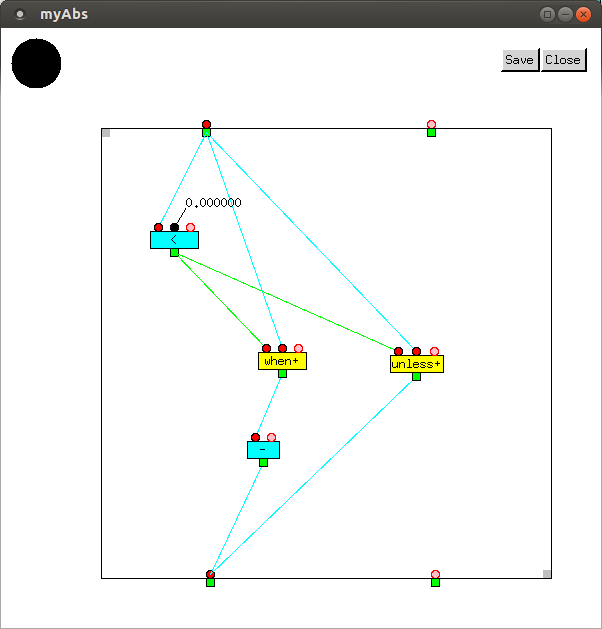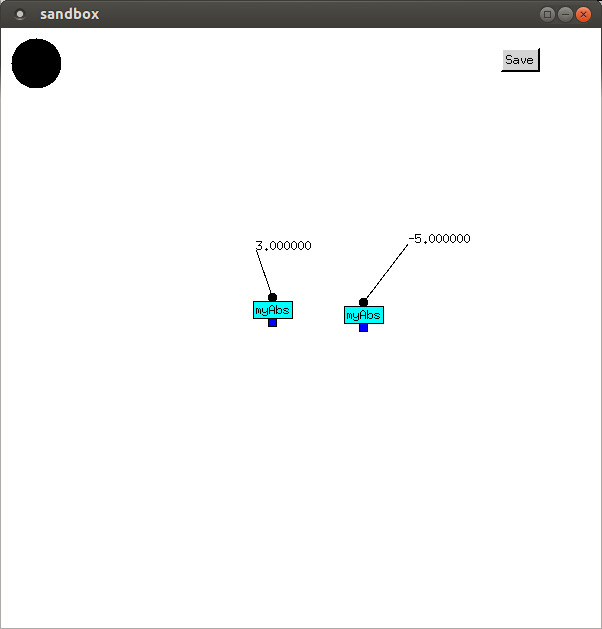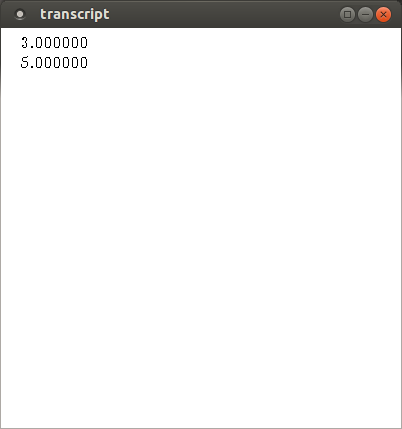# Conditional Dataflow

More general functions require conditional constructs. C and Java have `if` and `switch` statements, and `?:` expressions. Lisp has `cond`, `if`, `when`, `unless`, and `case` expressions. In C, Java, and Lisp, these alter the flow of control. Full Metal Jacket doesn't have flow of control, however. It has flow of data.

Conditional dataflow in Full Metal Jacket is done using `when+` and `unless+` vertices. Each takes a boolean value, and a number of other values, at its inputs. `when+` outputs the other values if the first is true (any value other than `NIL`), and outputs no values if it is false (`NIL`). `unless+` behaves in the opposite manner, outputting if its first value is `NIL`.

`myAbs` is a function which returns the absolute value of a `Real` number:Two separate calls were made, in the order shown in the transcript.

In the first call, the `<` vertex returned `NIL`, so the `when+` vertex did not transmit any value to the `-` vertex, which never executed. The `unless+` vertex transmitted `myAbs`'s input to the outgate.

In the second call, the `<` vertex returned `T`, so the `when+` vertex transmitted `myAbs`'s input to the `-` vertex, which negated it before transmitting it to the outgate. The `unless+` vertex did not transmit any value to the outgate.### Notation

Conditional vertices, as well as those used for iteration, are yellow. Incidentally, when `-` is given a single input, as is done here, it negates it; otherwise, it subtracts all other inputs from its first input.